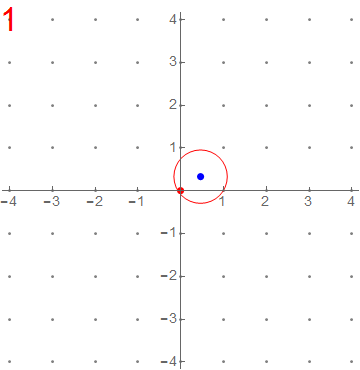## Circle enclosing N lattice points

14 May 2016

Did you know?
For every natural number N, there exists a circle enclosing exactly N lattice points!The above was posted on the Facebook group of Math n Physics Club of my institute. It triggered a discussion about the center of such a circle with one guy commenting without reason enough that it has to be an irrational point and any irrational point would do.

I found it interesting and thought over it while taking a shower. If the center is at origin, then this is impossible. If you try to enclose a point at a distance $\sqrt{d^2}$, you will find that $d^2 = x^2 + y^2$ where you don’t have a unique choice of $x$ and $y$.

Now note that if the center is a rational point, say $(\frac{a}{b},\frac{c}{d})$, then you can scale the space by $bd$, then the center lies at $(ad,bc)$ and then a simple translation will take you to origin and our hypothesis is reduced to the above case raising a contradiction against our assumption that the center is a rational point.

Thus, the center must be an irrational point. I do not know if any irrational point would do though.

My two cents!